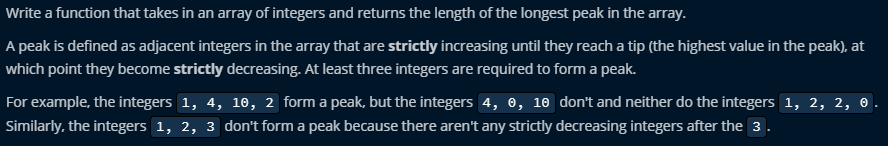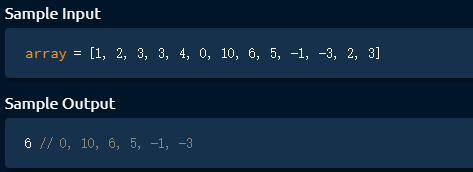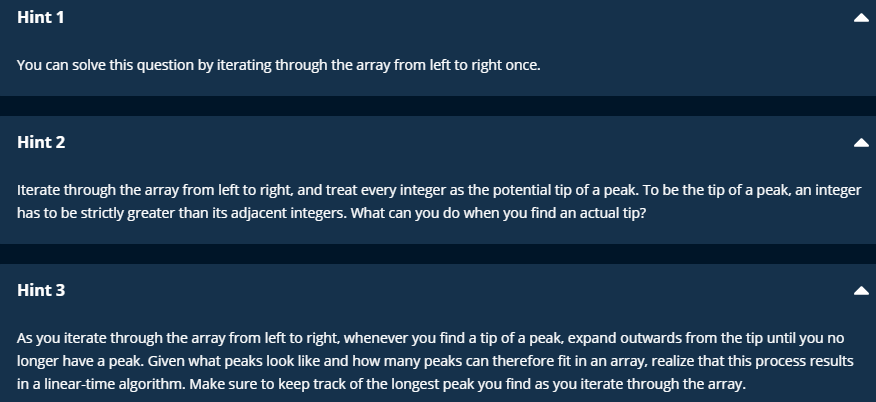5G时代下一个聚合的编程学习网

# Longest Peak

## Problem Statement## Sample## Analysis## Code

``` 1 def longestPeak(array):
2     # Write your code here.
3     longestPeakLength = 0
4     i = 1 # the first one(index 0) can not be the peak
5     while i < len(array) - 1: #check peak element
6         isPeak = array[i-1] < array[i] and array[i] > array[i+1]
7         if not isPeak:
8             i += 1
9             continue
10         leftIdx = i - 2 # expand the left side around peak
11         while leftIdx >=0 and array[leftIdx] < array[leftIdx + 1]:
12             leftIdx -= 1
13         rightIdx = i + 2# expand the right side around peak
14         while rightIdx < len(array) and array[rightIdx] < array[rightIdx - 1]:
15             rightIdx += 1
16
17         currentPeakLength = rightIdx - leftIdx - 1 # calculate the length of current peak
18         longestPeakLength = max(longestPeakLength, currentPeakLength) # update the longest length of peak
19         i = rightIdx # update i, inside the rightIdx will be the part of calculated peak, we don't need to recalculate
20     return longestPeakLength
21             ```

## Time and Space complexity

Time: O(N)-> outer loop. iterate the array once, inner loop, every element will be checked at most two or three times.(2N + 3N) -> O(N)

Space: O(1)